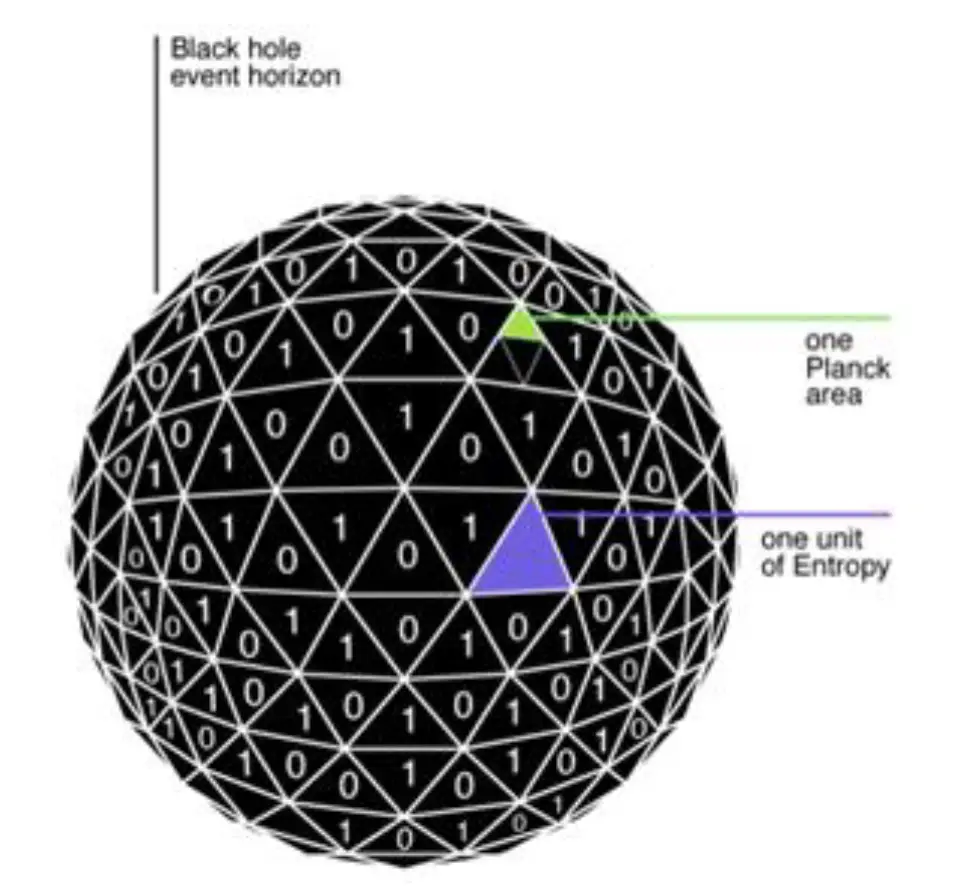# Black Hole thermodynamics

Understanding the ‘Law of Areas,’ in regards to the black hole entropy, based on an underlying fundamental theory has been one of the goals pursued by all models of quantum gravity.

However, until now, it was not known which microstates were counted by the entropy of black holes.

But following a thermodynamic analysis of black hole, as the archetypal quantum gravitational object, the black hole, accidentally turned out to just like a thermodynamic system.

We can say that there are fundamental degrees of freedom, considered as the minimum number of coordinates required to specify a configuration, that give rise to horizon temperature and entropy.

If we consider that the degree of freedom of a system can be viewed as the minimum number of coordinates required to specify a configuration. So YES ... W reflects the degree of freedom of a system.

Furthermore, generally, the entropy is proportional to the number of bits necessary to describe the state of the system considered.

This result, which was demonstrated by Jacob Bekenstein corresponds to the interpretation in terms of bits of information.

$$S_{BH}$$=$$k \over 4$$($$c^3 \over ħG$$)A=k$$A \over l^2_p$$=$$S=k ln(W)$$

SBH= Bekenstein-Hawking  entropy (black hole), KB=Boltzmann constant, c=speed of light,  ħ= Reduced Planck's constant, G=Gravitational Constant, A=area of the event horizon of the black hole, $$L^2_p$$= Planck surface, S= entropy Boltzmann,  W = microstatesThe thermodynamics of black holes suggests certain relationships between the entropy of black holes and their geometry

The Planck surface is a specific expression for black-hole entropy in terms of black-hole

The Planck surface intervenes in particular in the thermodynamics of black holes, where it corresponds to a quantity of information

Black hole as a thermodynamic system, point of view from Jacob Bekenstein saying in substance:

“Therefore, the decrease in area and entropy is of a statistical nature, and is quite analogous to the decrease in entropy of a thermodynamic system due to statistical fluctuations”.

About thermodynamic system in equilibrium we must think about local thermodynamic equilibrium. Where equilibrium does not require either local or global stationarity.

Each small locality must change slowly enough to practically sustain its local Maxwell–Boltzmann distribution of molecular velocities. Local thermodynamic equilibrium (LTE) means that those intensive parameters are varying in space and time, but are varying so slowly that, for any point, one can assume thermodynamic equilibrium in some neighborhood about that point. In other words, it doesn’t require that every small locality must have a constant temperature.

We see so that Black hole are subject to the Local thermodynamic equilibrium (LTE), in accordance with definition of the entropy concept from the theory of entropic information.